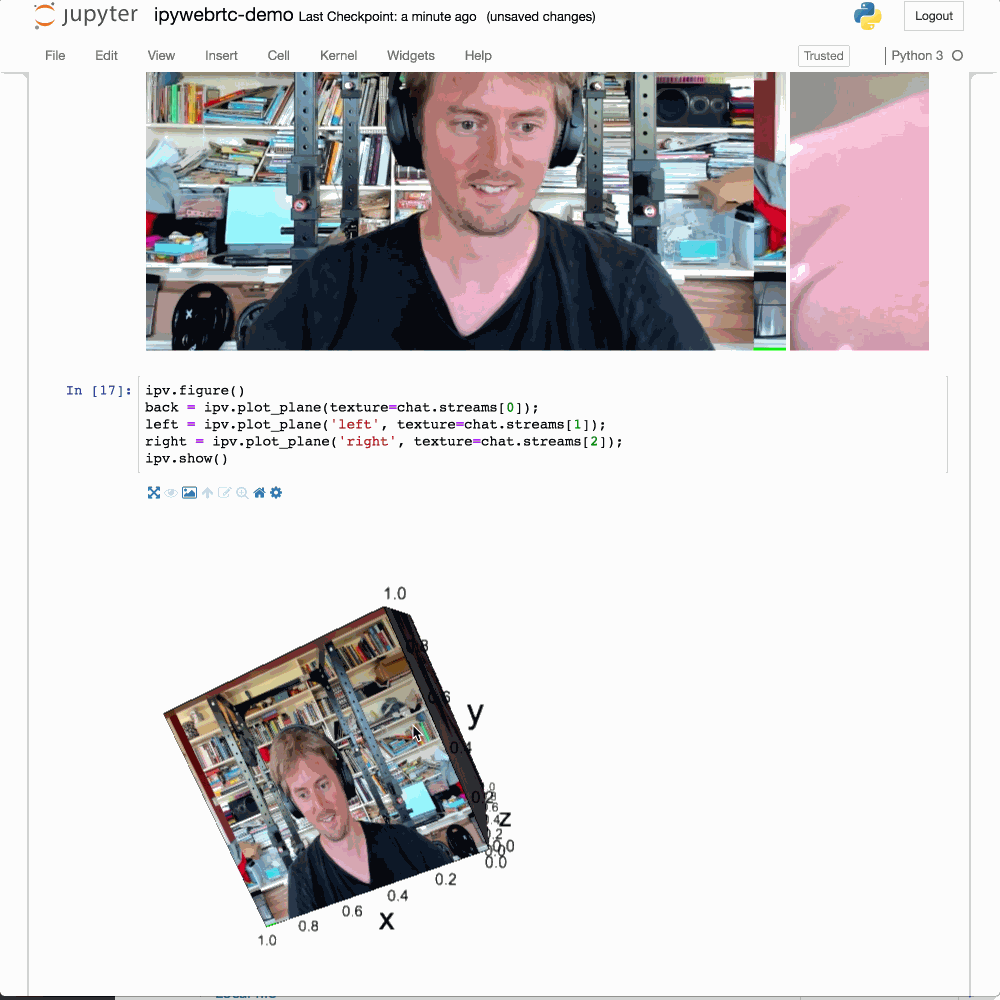# List of Extensions on Jupyter@JSC¶

If you want to use any of these extensions, feel free to use our examples as a starting point.

## AppMode (Classic Notebook only)¶

AppMode is a Jupyter extension that turns notebooks into web applications.
To use it click on the menu on "Help" -> "Launch Classic Notebook".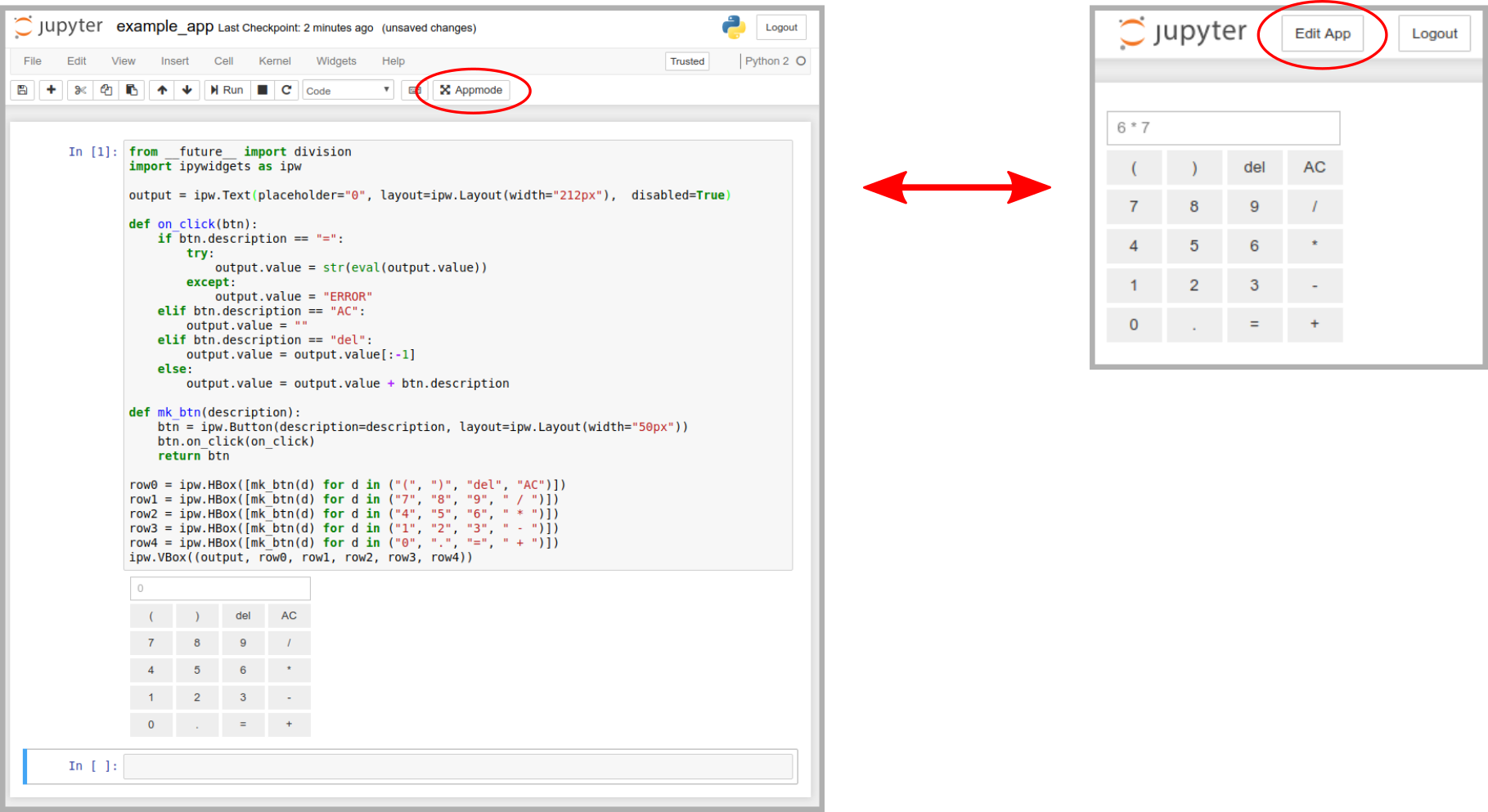## Celltags¶

The JupyterLab cell tags extension enables users to easily add, view, and manipulate descriptive tags for notebook cells.
The extension includes the functionality to select all cells with a given tag, supporting the performance of any operation on those cells.
Click on the wrench Symbol on the left sidebar to use it.## Codefolding (Classic Notebook only)¶

CodeFolding adds codefolding functionality from CodeMirror to a codecell.
To use it click on the menu on "Help" -> "Launch Classic Notebook".## Code Formatter¶

Code Formatter allows you to format a Notebook for.
You can use it as shown below or you click on "Format notebook" at the top of your notebook.## Dask (only on HPC Systems)¶

An extension to manage Dask clusters, as well as embed Dask's dashboard plots directly into JupyterLab panes.
Watch this video until the end to unterstand how to use Dask in JupyterLab. At the moment we only offer to use the panels inside of JupyterLab.
We have introduction notebooks for this extensions here (or open the gitlab extension on the left sidebar).

## Drawio¶

Drawio is a JupyterLab extension for standalone integration of drawio / mxgraph into jupyterlab.

There is a known save-bug in this extension. Please use the menu of JupyterLab to save files from this extension.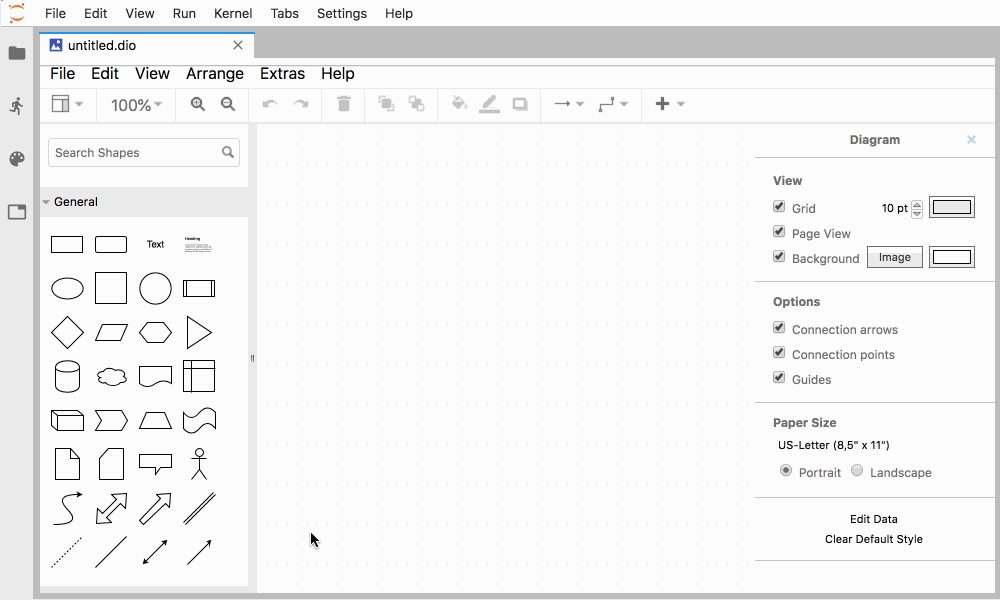## Git¶

A JupyterLab Git extension for version control using git.## Ipydatawidgets¶

Ipydatawidgets is a set of widgets to help facilitate reuse of large datasets across different widgets, and different packages.

In [ ]:
import numpy as np
from ipydatawidgets import DataImage
np.random.seed(0)
data = np.array(255 * np.random.rand(200, 200, 4), dtype='uint8')
DataImage(data=data)

In [ ]:
SIDELEN = 300
grad = np.linspace(0, 255, SIDELEN, dtype='uint8')
# red and green components:
# add blue and alpha components (zero and 255):
rgba = np.dstack([
rg,
np.zeros((SIDELEN, SIDELEN), dtype='uint8'),
255 * np.ones((SIDELEN, SIDELEN), dtype='uint8')
])
DataImage(data=rgba)


## IPyVolume¶

IPyvolume is a Python library to visualize 3d volumes and glyphs.

In [ ]:
import numpy as np
import ipyvolume as ipv
V = np.zeros((128,128,128))# our 3d array
# outer box
V[30:-30,30:-30,30:-30] = 0.75
V[35:-35,35:-35,35:-35] = 0.0
# inner box
V[50:-50,50:-50,50:-50] = 0.25
V[55:-55,55:-55,55:-55] = 0.0
ipv.quickvolshow(V, level=[0.25, 0.75], opacity=0.03, level_width=0.1, data_min=0, data_max=1)


## JupyterLab LaTeX Extension¶

The LaTeX Extension is an extension for JupyterLab which allows for live-editing of LaTeX documents.
Here you can find a short example.

## JupyterLab LMod¶

An extension that allows user to interact with environment modules before launching kernels.
To check the Pythonpath/PATH of your current kernel just run the following command. Remember to restart the kernel after loading other modules.

In [ ]:
!echo $PATH # or !echo$PYTHONPATHThe entries are clickable, and scroll the document to the heading in question.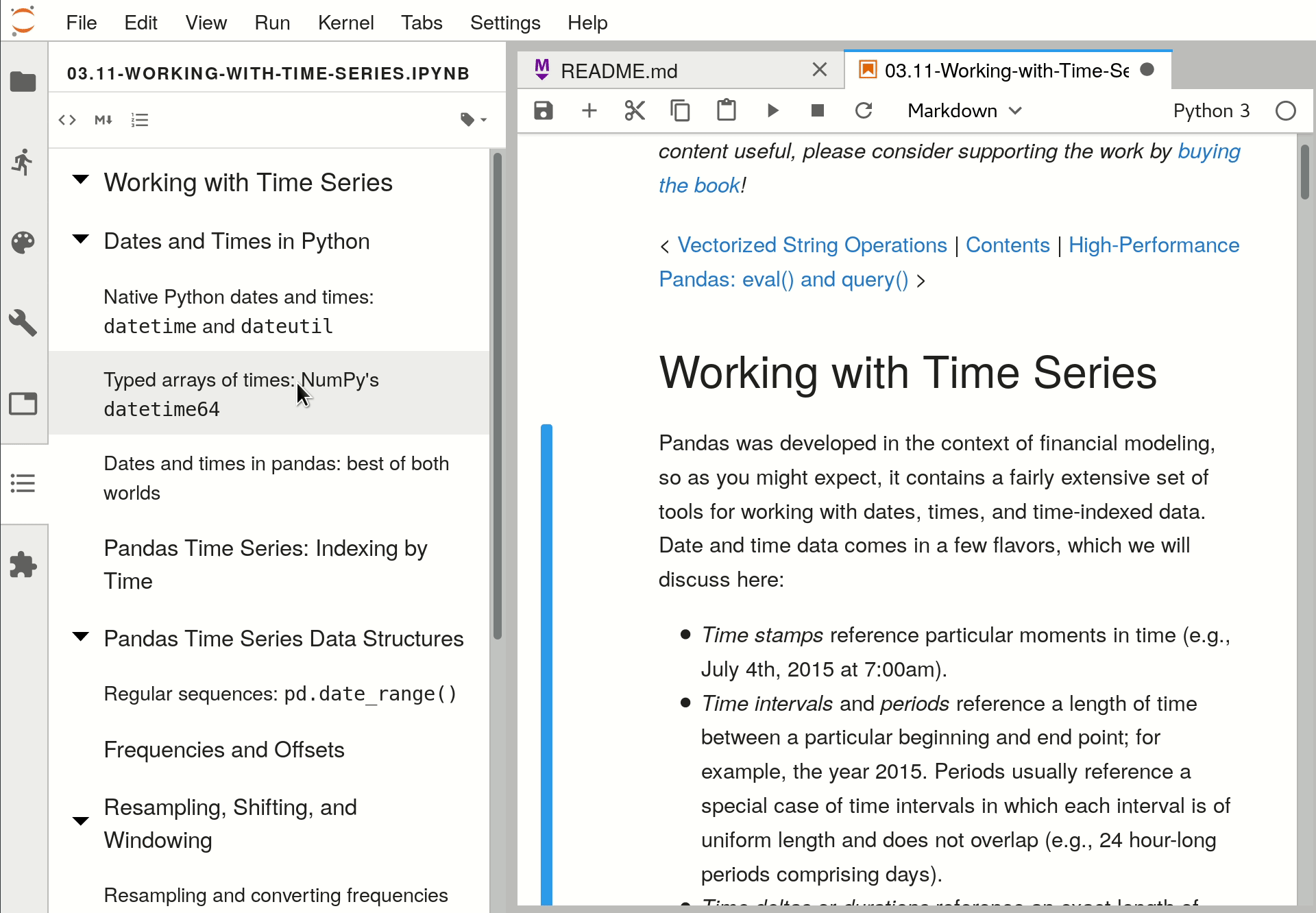## Jupyter ThreeJS¶

A Python / ThreeJS bridge utilizing the Jupyter widget infrastructure.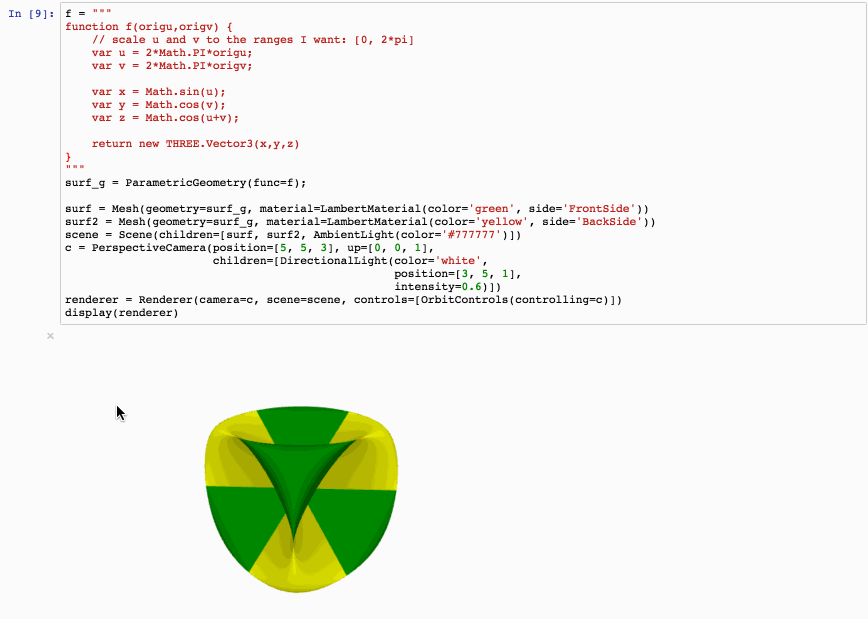## Leaflet¶

The Jupyterlab Leaflet extension enables interactive maps.
You can find several example notebooks here.## MatplotLib¶

MatplotLib enables the interactive features of matplotlib in Jupyterlab.
Besides, the figure canvas element is a proper Jupyter interactive widget which can be positioned in interactive widget layouts.

In [ ]:
# Enable the matplotlib widget
%matplotlib widget

In [ ]:
import matplotlib.pyplot as plt
from mpl_toolkits.mplot3d import axes3d

fig = plt.figure()

# Grab some test data.
X, Y, Z = axes3d.get_test_data(0.05)

# Plot a basic wireframe.
ax.plot_wireframe(X, Y, Z, rstride=10, cstride=10)

fig.canvas.layout.max_width = '800px'

plt.show()

In [ ]:
# When using the widget backend from ipympl,
# fig.canvas is a proper Jupyter interactive widget, which can be embedded in
# Layout classes like HBox and Vbox.

# One can bound figure attributes to other widget values.
import numpy as np
from ipywidgets import HBox, FloatSlider

plt.ioff()
plt.clf()

slider = FloatSlider(
orientation='vertical',
value=1.0,
min=0.02,
max=2.0
)

fig = plt.figure(3)

x = np.linspace(0, 20, 500)

lines = plt.plot(x, np.sin(slider.value  * x))

def update_lines(change):
lines.set_data(x, np.sin(change.new * x))
fig.canvas.draw()
fig.canvas.flush_events()

slider.observe(update_lines, names='value')

HBox([slider, fig.canvas])


## NBDime¶

NBDime provides tools for diffing and merging of Jupyter Notebooks.

## NBExtensions Configurator (Classic Notebook only)¶

A server extension for jupyter notebook which provides configuration interfaces for notebook extensions (nbextensions).
Works only with the classic notebook, not with JupyterLab.
To use it click on the menu on "Help" -> "Launch Classic Notebook".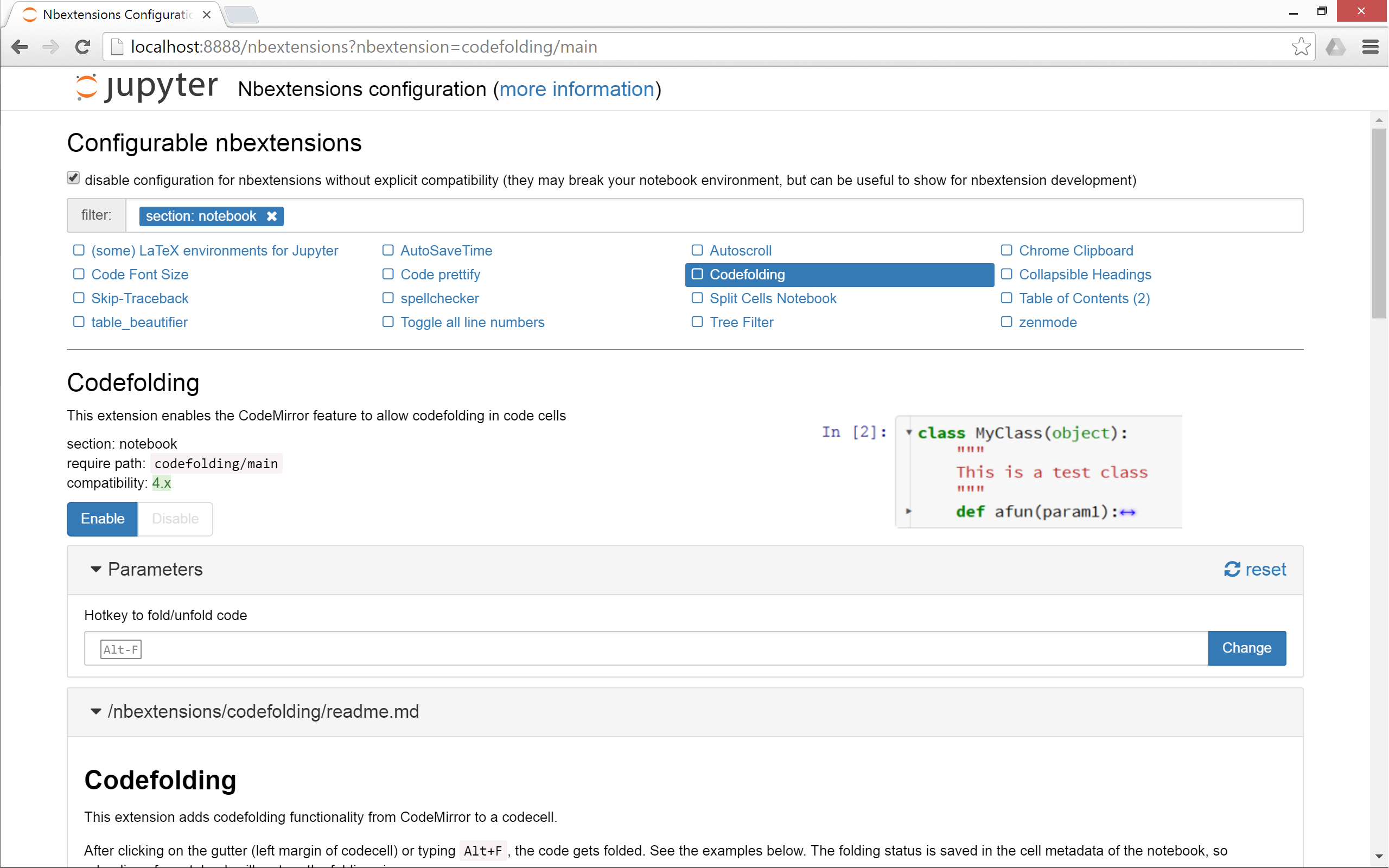## Plotly¶

A JupyterLab extension for plotly

In [ ]:
import plotly
from plotly.offline import init_notebook_mode, iplot
init_notebook_mode(connected=True)
trace0 = plotly.graph_objs.Scatter(
x=[1, 2, 3, 4],
y=[10, 15, 13, 17]
)
trace1 = plotly.graph_objs.Scatter(
x=[1, 2, 3, 4],
y=[16, 5, 11, 9]
)

iplot([trace0, trace1])


## Quick Open¶

Quick Open allows you to quickly open a file in JupyterLab by typing part of its name. Just click on the lens symbol at the left sidebar.
Takes a long time on HPC systems.## RISE (Classic Notebook only)¶

With RISE, a Jupyter notebook extension, you can instantly turn your jupyter notebook into a live reveal.js-based presentation.
To use it click on the menu on "Help" -> "Launch Classic Notebook".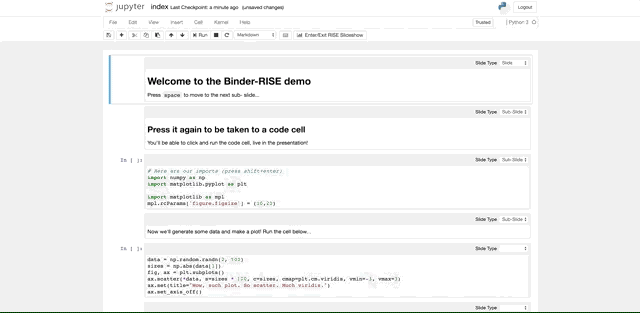## Sidecar¶

A sidecar output widget for JupyterLab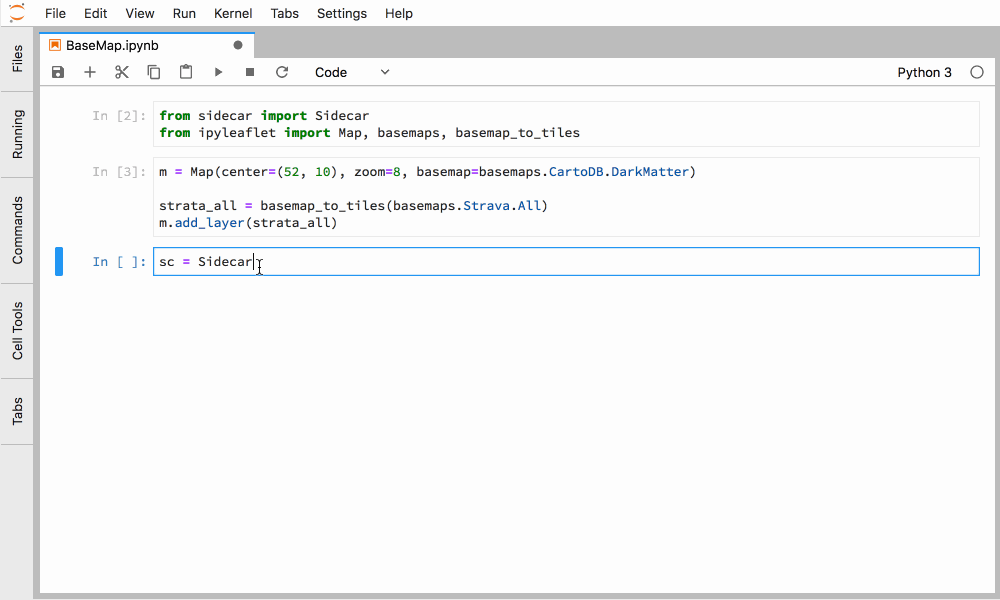## Voilà Preview¶

Voilà turns Jupyter notebooks into standalone web applications.

Unlike the usual HTML-converted notebooks, each user connecting to the Voilà tornado application gets a dedicated Jupyter kernel which can execute the callbacks to changes in Jupyter interactive widgets.

This extension allows you to render a Notebook with Voilà, so you can see how your Notebook will look with it.

 \$ wget --no-check-certificate https://jupyter-jsc.fz-juelich.de/static/files/voila_basics.ipynb